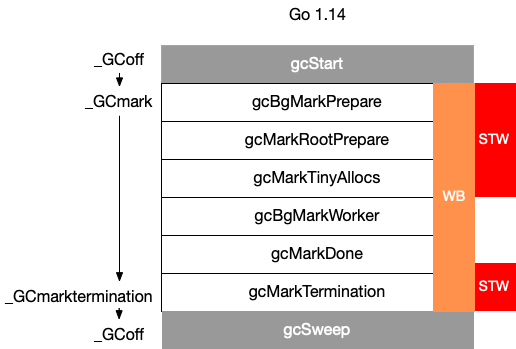# GC 的实现细节 #

## 10. Go 语言中 GC 的流程是什么？ #

SweepTermination 清扫终止阶段，为下一个阶段的并发标记做准备工作，启动写屏障 STW
Mark 扫描标记阶段，与赋值器并发执行，写屏障开启 并发
MarkTermination 标记终止阶段，保证一个周期内标记任务完成，停止写屏障 STW
GCoff 内存清扫阶段，将需要回收的内存归还到堆中，写屏障关闭 并发
GCoff 内存归还阶段，将过多的内存归还给操作系统，写屏障关闭 并发## 11. 触发 GC 的时机是什么？ #

Go 语言中对 GC 的触发时机存在两种形式：

1. 主动触发，通过调用 runtime.GC 来触发 GC，此调用阻塞式地等待当前 GC 运行完毕。

2. 被动触发，分为两种方式：

• 使用系统监控，当超过两分钟没有产生任何 GC 时，强制触发 GC。

• 使用步调（Pacing）算法，其核心思想是控制内存增长的比例。• 设第 n 次触发 GC 时 (n > 1)，估计得到的堆增长率为 $h_t^{(n)}$、运行过程中的实际堆增长率为 $h_a^{(n)}$，用户设置的增长率为 $\rho = \text{GOGC}/100$（ $\rho > 0$）则第 $n+1$ 次出触发 GC 时候，估计的堆增长率为：

$$h_t^{(n+1)} = h_t^{(n)} + 0.5 \left[ \frac{H_g^{(n)} - H_a^{(n)}}{H_a^{(n)}} - h_t^{(n)} - \frac{u_a^{(n)}}{u_g^{(n)}} \left( h_a^{(n)} - h_t^{(n)} \right) \right]$$

• 特别的，$h_t^{(1)} = 7 / 8$，$u_a^{(1)} = 0.25$，$u_g^{(1)} = 0.3$。第一次触发 GC 时，如果当前的堆小于 $4\rho$ MB，则强制调整到 $4\rho$ MB 时触发 GC

• 特别的，当 $h_t^{(n)}<0.6$时，将其调整为 $0.6$，当 $h_t^{(n)} > 0.95 \rho$ 时，将其设置为 $0.95 \rho$

• 默认情况下，$\rho = 1$（即 GOGC = 100），第一次触发 GC 时强制设置触发第一次 GC 为 4MB，可以写如下程序进行验证：

  1 2 3 4 5 6 7 8 9 10 11 12 13 14 15 16 17 18 19 20 21 22 23 24 25 26 27 28 29 30 31 32 33 34 35 36 37 38 39 40 41  package main import ( "os" "runtime" "runtime/trace" "sync/atomic" ) var stop uint64 // 通过对象 P 的释放状态，来确定 GC 是否已经完成 func gcfinished() *int { p := 1 runtime.SetFinalizer(&p, func(_ *int) { println("gc finished") atomic.StoreUint64(&stop, 1) // 通知停止分配 }) return &p } func allocate() { // 每次调用分配 0.25MB _ = make([]byte, int((1<<20)*0.25)) } func main() { f, _ := os.Create("trace.out") defer f.Close() trace.Start(f) defer trace.Stop() gcfinished() // 当完成 GC 时停止分配 for n := 1; atomic.LoadUint64(&stop) != 1; n++ { println("#allocate: ", n) allocate() } println("terminate") } 

$go build -o main$ GODEBUG=gctrace=1 ./main
#allocate:  1
(...)
#allocate:  20
gc finished
gc 1 @0.001s 3%: 0.016+0.23+0.019 ms clock, 0.20+0.11/0.060/0.13+0.22 ms cpu, 4->5->1 MB, 5 MB goal, 12 P
scvg: 8 KB released
scvg: inuse: 1, idle: 62, sys: 63, released: 58, consumed: 5 (MB)
terminate


gc 1 @0.001s 3%: 0.016+0.23+0.019 ms clock, 0.20+0.11/0.060/0.13+0.22 ms cpu, 4->5->1 MB, 5 MB goal, 12 P

1. 程序在完成第一次 GC 后便终止了程序，符合我们的设想
2. 第一次 GC 开始时的堆大小为 4MB，符合我们的设想
3. 当标记终止时，堆大小为 5MB，此后开始执行清扫，这时分配执行到第 20 次，即 20*0.25 = 5MB，符合我们的设想

 1 2 3 4  for n := 1; n < 50; n++ { println("#allocate: ", n) allocate() } 

$go build -o main$ GODEBUG=gctrace=1,gcpacertrace=1 ./main
#allocate:  1
(...)

pacer: H_m_prev=2236962 h_t=+8.750000e-001 H_T=4194304 h_a=+2.387451e+000 H_a=7577600 h_g=+1.442627e+000 H_g=5464064 u_a=+2.652227e-001 u_g=+3.000000e-001 W_a=152832 goalΔ=+5.676271e-001 actualΔ=+1.512451e+000 u_a/u_g=+8.840755e-001
#allocate:  28
gc 1 @0.001s 5%: 0.032+0.32+0.055 ms clock, 0.38+0.068/0.053/0.11+0.67 ms cpu, 4->7->3 MB, 5 MB goal, 12 P

(...)
#allocate:  37
pacer: H_m_prev=3307736 h_t=+6.000000e-001 H_T=5292377 h_a=+7.949171e-001 H_a=5937112 h_g=+1.000000e+000 H_g=6615472 u_a=+2.658428e-001 u_g=+3.000000e-001 W_a=154240 goalΔ=+4.000000e-001 actualΔ=+1.949171e-001 u_a/u_g=+8.861428e-001
#allocate:  38
gc 2 @0.002s 9%: 0.017+0.26+0.16 ms clock, 0.20+0.079/0.058/0.12+1.9 ms cpu, 5->5->0 MB, 6 MB goal, 12 P


• 第一次估计得到的堆增长率为 $h_t^{(1)} = 0.875$
• 第一次的运行过程中的实际堆增长率为 $h_a^{(1)} = 0.2387451$
• 第一次实际的堆大小为 $H_a^{(1)}=7577600$
• 第一次目标的堆大小为 $H_g^{(1)}=5464064$
• 第一次的 CPU 实际使用率为 $u_a^{(1)} = 0.2652227$
• 第一次的 CPU 目标使用率为 $u_g^{(1)} = 0.3$

$$h_t^{(2)} = h_t^{(1)} + 0.5 \left[ \frac{H_g^{(1)} - H_a^{(1)}}{H_a^{(1)}} - h_t^{(1)} - \frac{u_a^{(1)}}{u_g^{(1)}} \left( h_a^{(1)} - h_t^{(1)} \right) \right]$$

$$= 0.875 + 0.5 \left[ \frac{5464064 - 7577600}{5464064} - 0.875 - \frac{0.2652227}{0.3} \left( 0.2387451 - 0.875 \right) \right]$$

$$\approx 0.52534543909$$

## 12. 如果内存分配速度超过了标记清除的速度怎么办？ #

 1 2 3 4 5 6 7 8  func mallocgc(t typ.Type, size uint64) { if enableMarkAssist { // 进行标记辅助，此时用户代码没有得到执行 (...) } // 执行内存分配 (...) }